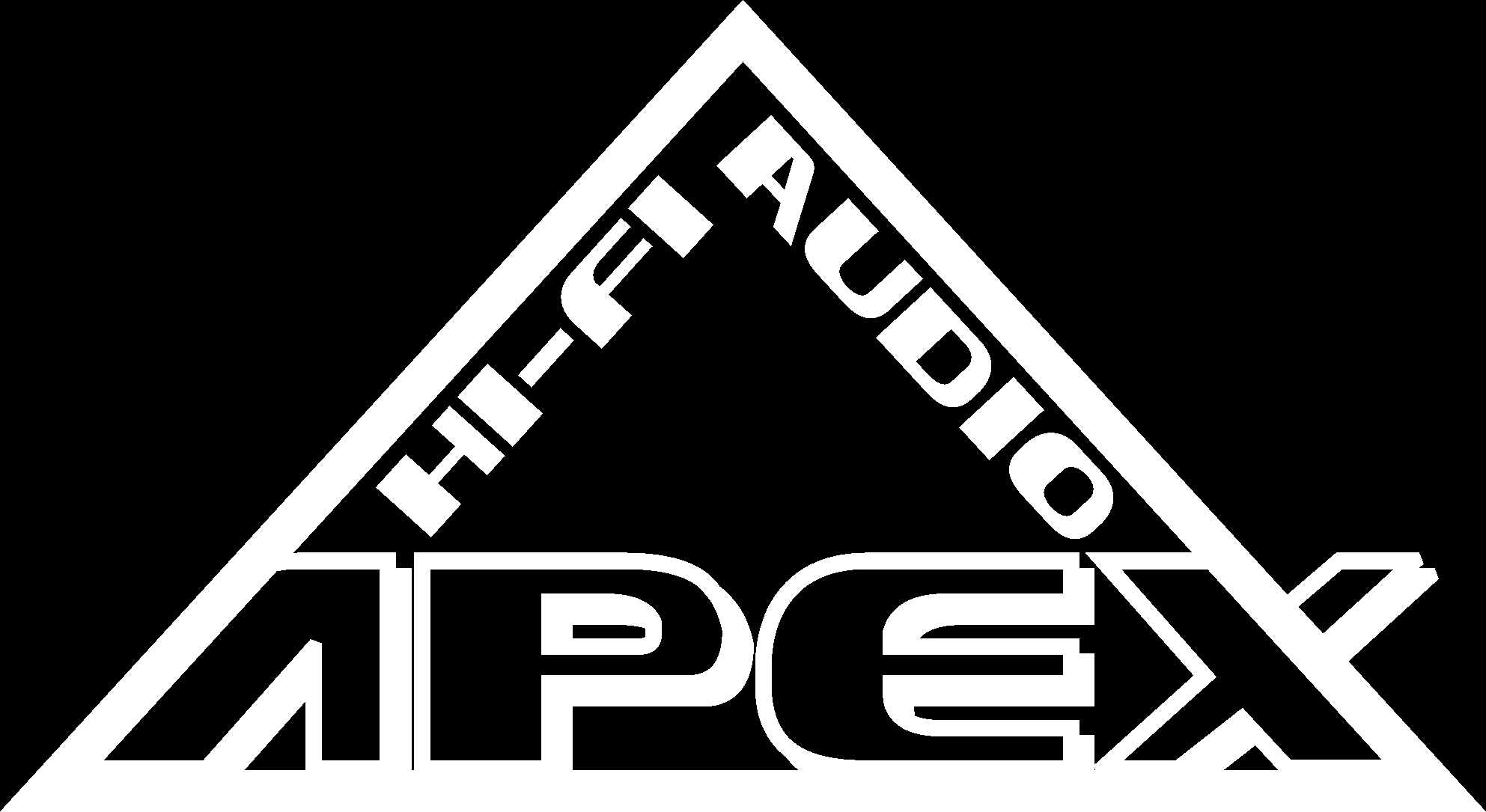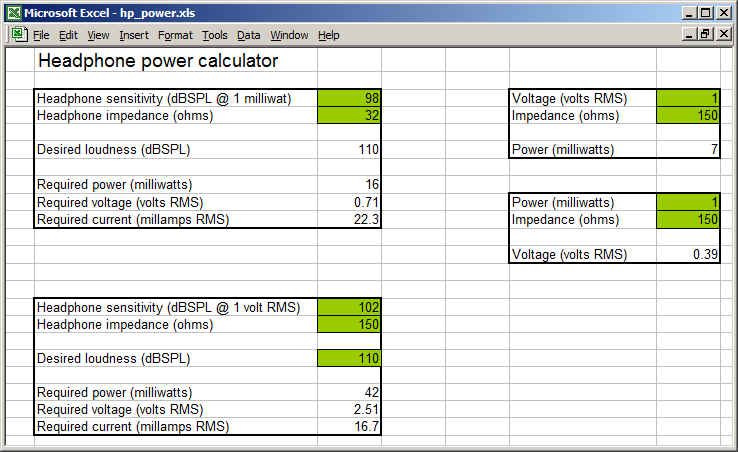backHeadphone and amplifier specs - power, voltage, dBSPL...

There seems to be a lot of confusion about how much power or voltage is needed to drive headphones.  We'll try and explain what all the numbers mean, and how to tell how much of an amplifier you need to drive a given set of headphones.

TOO LOUD WILL HURT YOU!

There is a real danger of permanently damaging your hearing while listening to headphones.  Before you obsess about having a sound system that will get loud enough to make your ears bleed or reproduce the sound of sitting in front of the speaker stacks at a Kiss concert, please have some common sense, and learn about the dangers of hearing loss from listening to music too loud.  There are many web resources to learn about hearing damage... here is one run by Oregon health Sciences University (OHSU) that explains things in an easy-to-understand way:  Dangerous Decibels.  Also visit the NIH's Wise Ears site.

Sound Pressure Level, or dBSPL

Let's start with the basics: Loudness, or sound pressure level, is measured in units call decibels.  This is usually abbreviated dBSPL.  dBSPL is a logarithmic scale; 10x the loudness is 10 dBSPL higher... 100x is 20 dBSPL higher... etc.

To give you a reference of what these numbers mean, here's a table listing some common sounds, and the approximate sound pressure level in dBSPL:

 Sound dBSPL Library 30 dB Normal conversation at 1 meter 40 - 60 dB Car driving by at 10 meters 60 - 80 dB Busy city traffic 90 dB Jackhammer at 1 meter 100 dB Typical club concert 110 dB Threshold of pain 130 dB Jet engine at 30 meters 150dB

It's generally agreed that levels over 120 dBSPL for short periods, or over 85 dBSPL for  extended periods of time, will cause hearing damage. 100 - 110 dBSPL is about as loud as most people can stand to listen for any length of time.  Most people listen to music at home below 85 dBSPL.

There are two headphone specifications that are important when selecting a headphone amplifier.  These specs are the sensitivity and the impedance.

Sensitivity is rated in dBSPL at a particular input signal level.  This level is usually specified at 1 milliwatt (1/1000th of a watt).  Sometimes it is specified at 1V RMS.  1 milliwatt is a POWER level... 1 volt is a VOLTAGE level.   If you know the impedance of the headphones, you can translate power (watts) into voltage (volts), and vice-versa.  The equation to calculate power is P = E2 / R, where P is power, E is voltage, and R is impedance.  So you can see that power is proportional to the square of voltage.

Loudness is also proportional to power.  So if you double the power, you also double the sound pressure level (dBSPL).

To determine how loud a headphone is - that is, how many dBSPL it is producing - at a given power level, you can use the headphone specification at 1 milliwatt and a little math.  Let's use an example:

Let's say we have a pair of headphones that is specified at 100 dBSPL at 1 milliwatt.  That means (as you might guess!) if you apply a 1 milliwatt signal, the headphones will produce 100 dBSPL.   Each time you multiply the power by 10, you increase the sound pressure level by 10 dB.  So 10 milliwatts into this headphone would give you 110 dBSPL... 100 milliwatts would be 120 dBSPL... 1 watt would be 130 dBSPL...

In equation form, dBSPL at power P (in milliwatts) is given by:  dBSPL =  dB(1mW) + 10 * log10 (P)

About that power level... The power delivered to a load, as shown above, depends on the voltage applied (E) and the impedance (R).  Impedance is rated in ohms.   But unlike speakers, which are almost all between 4 and 8 ohms, headphones are all over the map, from 16 ohms to as much as 600 ohms.  Delivering 1 milliwatt of power into 16 ohms takes 126 millivolts... but delivering that power into 600 ohms requires 775 millivolts.  So you can see that the VOLTAGE needed to drive a headphone to a given loudness depends not only on the sensitivity of the headphones, but also the IMPEDANCE of the headphones.

Unlike speaker amplifiers which are rated using power (watts into 8 ohms), headphone amplifiers are usually rated by how much output VOLTAGE they can deliver, not how much POWER they can deliver.  This is done for a number of reasons - partly because it is easier, and partly because it does not depend much on the headphone impedance.

In reality, headphone amplifiers also have a maximum amount of CURRENT that they can deliver.  This is often the limiting factor for how much power a headphone amplifier can deliver into a low-impedance load, say, 50 ohms or lower.  Power can also be calculated as P = I2 * R, where  I is current (and R is impedance again).

To simplify all this, it's common to specify the maximum output of a headphone amp in volts, at two different load impedances, like 150 ohms and 32 ohms.  For most headphone amps, a 32 ohm load is often limited by the maximum amount of CURRENT the amp can put out before distorting, while the maximum output voltage into 150 ohms is limited by the maximum amount of VOLTAGE that the amp can put out.  So for a given sound pressure level, you really need to calculate what voltage is needed, and what current is needed, and make sure the amp can deliver both.

Just to confuse you even more, some manufacturers state output voltage in PEAK, or PEAK-TO-PEAK volts, rather than RMS volts.  (RMS means root-mean-squared, and is representative of the actual power being delivered to the load).  You can translate peak volts to RMS volts by dividing by the square root of 2 (1.414); peak-to-peak can be translated to RMS by dividing by 2.828.

ACK! What does all this mean?

OK, let's try a real world example.  Let's say you have some Grado PS1000 headphones and you want to know how loud the Apex Peak will drive them.  The Grados are rated at 98 dBSPL @ 1 milliwatt, with an impedance of 32 ohms.  The peak is rated at 5.6V RMS into 33 ohms (close enough to 32 ohms).  First, we can determine the power that 5.6V will deliver into 342 ohms by E2/R, or 5.62/32, or 980 milliwatts.

To determine the loudness level, plug this into the equation above: dBSPL =  dB(1mW) + 10 * log10 (P), or 98 + 10 *  log10 (980), which equals 127.9 dBSPL.  Roughly the threshold of pain, and more than you should ever listen to.

You can also work backwards from a given loudness level to determine the power... and voltage... that you need to drive a given set of headphones.  this time let's take the HiFiMan HE-6 headphones, which are pretty inefficient at 83.5dB SPL @ 1 milliwatt, with an impedance of 50 ohms.  Let's say you want to be able to drive 110 dBSPL into these headphones, about what you would hear at a typical rock concert.  If we re-arrange the equation above, P = 10 (( dBSPL - dB(1mw) ) /10 )... so P = 10 (( 110 - 83.5 ) /10 ), or 446 milliwatts of power.  To convert this to voltage, re-arranging the power equation above, E = sqrt( P * R ), or sqrt (0.446 * 50), which equals 4.7V RMS.  You can also calculate the current... I = sqrt (P / R), or sqrt (0.446 / 50) or 95mA RMS.  (Note that ALL of the Apex amps can deliver more than this amount of power into 50 ohms).

For those of you mathematically challenged... or just lazy like me... here's a little spreadsheet to help you calculate this.  There are two calculators, one for headphones rated at 1 milliwatt, and one for those rated at 1V RMS (Sennheiser has started doing this).  Also, calculators to get power from voltage & impedance, or voltage from power & impedance.  You can enter data in the green cells... the results will show in the white ones.

Click on the image below to download the spreadsheet (sorry, it did not want to run inside the web page).And some reference headphone data I've gathered from the web:

 Manufacturer Model Efficiency @ 1mW (dB SPL) Impedance (ohms) AKG K701 105 62 AKG K1000 74 120 HiFiMan HE-6 83.5 50 HiFiMan HE-5 87.5 38 Sennheiser HD800 102 @ 1V* 300 Sennheiser HD650 103 @ 1V* 300 Sennheiser HD600 97 300 Grado SR60i 98 32 Grado SR225si 98 32 Grado RS1 98 32 Grado PS1000 98 32 Beyer T1 102 600 Beyer T5 102 32 Beyer DT880 96 32, 250, or 600

* Rated at 1V instead of 1mW